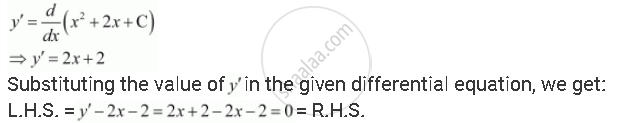Share

Verify that the Given Functions (Explicit Or Implicit) is a Solution of the Corresponding Differential Equation Y = X2 + 2x + C : Y′ – 2x – 2 = 0 - CBSE (Science) Class 12 - Mathematics

ConceptGeneral and Particular Solutions of a Differential Equation

Question

verify that the given functions (explicit or implicit) is a solution of the corresponding differential equation

y = x2 + 2x + C  :  y′ – 2x – 2 = 0

Solution

y = x2 + 2x + C

Differentiating both sides of this equation with respect to x, we get:Hence, the given function is the solution of the corresponding differential equation.

Is there an error in this question or solution?

APPEARS IN

NCERT Solution for Mathematics Textbook for Class 12 (2018 to Current)
Chapter 9: Differential Equations
Q: 2 | Page no. 385

Video TutorialsVIEW ALL 

Solution Verify that the Given Functions (Explicit Or Implicit) is a Solution of the Corresponding Differential Equation Y = X2 + 2x + C : Y′ – 2x – 2 = 0 Concept: General and Particular Solutions of a Differential Equation.
S